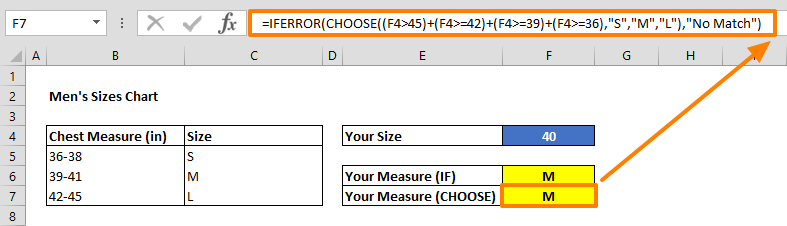A popular use case for the IF function is creating nested formulas that can check for various criteria. However, nested IF statements can get pretty complicated and cumbersome when dealing with several conditions. In this article, we’re going to show you how to use the CHOOSE function as an Excel nested IF alternative, and take a look at the differences between these two Excel case statements, and how you can compare several conditions more efficiently. You can download our sample workbook below.

## The Basics of the IF Function

The IF function is one of the most popular functions in Excel, as it can create logic-based conditions. The IF function gives a TRUE or FALSE result depending on the outcome of the condition check.

There really are no other alternatives to the IF function, and that's why Excel users typically prefer nested IF structures which means using the function over and over again. Let’s see how this works on an example. Below is a set of IF formulas inside one another.

=IF(F4>45,"No Match",IF(F4>=42,"L",IF(F4>=39,"M",IF(F4>=36,"S","No Match"))))

This formula checks 4 conditions, “S”, “M”, “L” and “other” to assign a measurement value. To do this, we need 2 extra IF functions which are connected to the negative result argument of the previous one. The idea here is following the rest of the logic tree, if first condition is not successful. Then look at the second condition, and continue until a satisfactory result is foind.As you can imagine, nested IF statements become harder to read and maintain as the number of condition increases. The CHOOSE function is essentially a more user-friendly alternative for these types of scenarios.

## Excel Nested IF Alternative: CHOOSE

The CHOOSE function returns a value from the list of arguments based on the specified position. Although, this definition doesn't seem related with what nested-ifs do, you can alter its use in this way. Let's see the syntax of the CHOOSE function:

### Syntax

CHOOSE(index_num, value1, [value2], …)

 index_num A number indicates which valueN argument will be returned. If index_num is 1, the CHOOSE returns value1; if it is 3, the CHOOSE returns value3; and so on. If index_num has decimal digits, Excel truncates the number. value1, [value2], … Values to return. Only required argument is value1. You can set up to 254 values.

### Sample

The following formula returns "Iyvsaur" string value because the index_num is 2 and the second item is "Iyvsaur":

=CHOOSE(2,"Bulbasaur","Ivysaur","Venusaur") returns "Ivysaur"

## Using CHOOSE Instead of Nested IFs

The CHOOSE function is a good alternative for generating an index number which points to the condition met. When Excel evaluates a condition, it returns a Boolean value based on the outcome - TRUE if condition is met, FALSE otherwise. For example,

=40>=39 returns TRUE

In addition to this, Excel also considers Boolean values to be 1 and 0 if they are in a mathematical operation. For example, the following formula returns 2 because the sum of FALSE, TRUE and TRUE values is equal to 0 + 1 + 1.

=(40>=42)+(40>=39)+(40>=36) returns 2

We can use this behavior to generate an index number (index_num) for the CHOOSE function. If there are 2 conditions are successful like previous example, the second value (value2) returns from the CHOOSE function.

Let's apply this to our use case:

=CHOOSE((F4>45)+(F4>=42)+(F4>=39)+(F4>=36),"S","M","L")

Note that, we list value arguments from the smallest to the largest, but they are listed in descending order for the nested if counterpart.

We recommend wrapping the formula inside an IFERROR function to handle undefined conditions.

=IFERROR(CHOOSE((F4>45)+(F4>=42)+(F4>=39)+(F4>=36),"S","M","L"),"No Match")## CHOOSE vs. IF

The advantage is the number of formulas used in this argument. When creating a nested IF statement, you need to be actively tracing where you’re at in the formula steps. Using the CHOOSE formula, you can do what 126 IF functions could, using a single function. You might also want to use the IFERROR function to cover not listed conditions.

Note: It is not recommended to create a IF function that contains 126 value-result pairs. Instead, try using the VLOOKUP function for matching large condition sets. For more information about the VLOOKUP, check out our HOW TO VLOOKUP article.